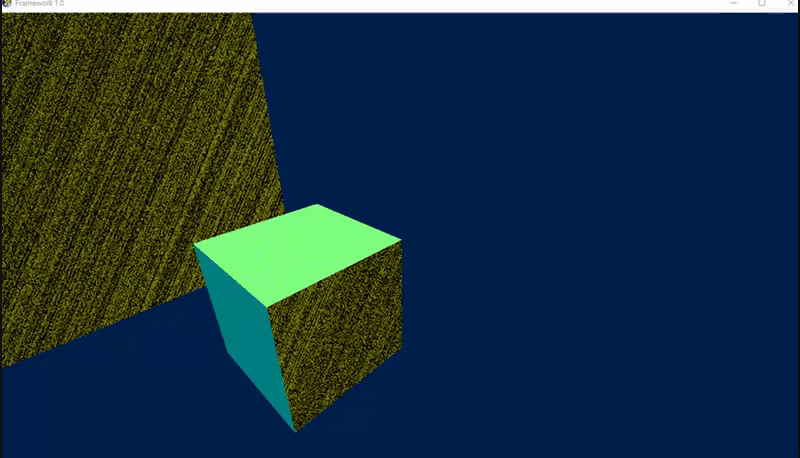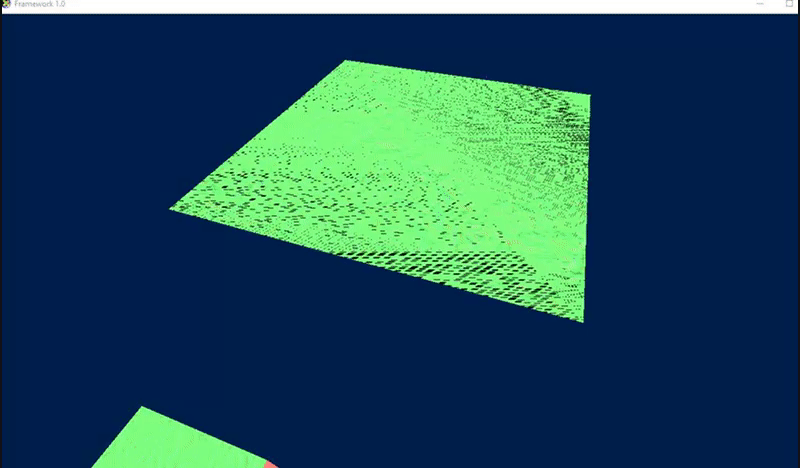# Per pixel normal mapping resulting in static on normals starting on Z axis

For the past week I've been trying to implement bump mapping in DirectX 11 using the Frank Luna's '3D Game Programming with DirectX 11' book. I got it working until I tried to add a flat 2D plane facing in the -Z axis and the texture appeared distorted. After a while I found out it was the bumped normals that were causing it.

I've used Terathon to calculate tangents after generating a mesh.

My code is as follows:

void AddTangentToVertexArray(const UINT vertexCount, const UINT indexCount, PackedVertex* vertices, const WORD* indices)
{
const UINT triangleCount = indexCount/ 3;

XMVECTOR* tan1 = new XMVECTOR[indexCount* 2];
XMVECTOR* tan2 = tan1 + indexCount;
ZeroMemory(tan1, indexCount* sizeof(XMVECTOR) * 2);

for (UINT a = 0; a < indexCount; a++)
{
WORD i1 = indices[(a * 3)];
WORD i2 = indices[(a * 3)+1];
WORD i3 = indices[(a * 3)+2];

const XMFLOAT3& v1 = vertices[i1].vertex;
const XMFLOAT3& v2 = vertices[i2].vertex;
const XMFLOAT3& v3 = vertices[i3].vertex;

const XMFLOAT2& w1 = vertices[i1].uv;
const XMFLOAT2& w2 = vertices[i2].uv;
const XMFLOAT2& w3 = vertices[i3].uv;

FLOAT x1 = v2.x - v1.x;
FLOAT x2 = v3.x - v1.x;
FLOAT y1 = v2.y - v1.y;
FLOAT y2 = v3.y - v1.y;
FLOAT z1 = v2.z - v1.z;
FLOAT z2 = v3.z - v1.z;

FLOAT s1 = w2.x - w1.x;
FLOAT s2 = w3.x - w1.x;
FLOAT t1 = w2.y - w1.y;
FLOAT t2 = w3.y - w1.y;

FLOAT r = 1.0f / (s1 * t2 - s2 * t1);
XMVECTOR sdir{ (t2 * x1 - t1 * x2) * r, (t2 * y1 - t1 * y2) * r,
(t2 * z1 - t1 * z2) * r };
XMVECTOR tdir{ (s1 * x2 - s2 * x1) * r, (s1 * y2 - s2 * y1) *r,
(s1 * z2 - s2 * z1) * r };

tan1[i1] += sdir;
tan1[i2] += sdir;
tan1[i3] += sdir;

tan2[i1] += tdir;
tan2[i2] += tdir;
tan2[i3] += tdir;
}

for (UINT a = 0; a < vertexCount; a++)
{
const XMVECTOR& t = tan1[a];

XMStoreFloat4(&vertices[a].tangent, XMVector3Normalize((t - n * XMVector3Dot(n, t))));

vertices[a].tangent.w = (XMVector3Less(XMVector3Dot(XMVector3Cross(n, t), tan2[a]), XMVECTOR{ 0.0f, 0.0f, 0.0f, 0.0f })) ? -1.0f : 1.0f;
}

delete[] tan1;
}


And I used Frank Luna's implementation to use those tangents to calculate the bitangent but in the the newer version of directX 11

float3 NormalSampleToWorldSpace(float3 normalMapSample, float3 NormalW, float4 TangentW)
{
float3 NormalT = 2.0f*normalMapSample - 1.0f;
float3 N = normalize(NormalW);
float3 T = normalize(TangentW - dot(TangentW, N)*N);
float3 B = cross(N, T) *TangentW.w;

float3x3 TBN = float3x3(T, B, N);

float3 bumpedNormalW = mul(NormalT, TBN);

return normalize(bumpedNormalW);
}


EDIT: After a couple hours of debugging I've found that changing the T calculation to float3 T = normalize(TangentW - dot(TangentW, N));// *N); seems to have stopped any distortions. If someone can confirm that this isn't going to cause later issues I'm willing to say it's solved.

All surfaces are using the same normal map in the following gifs.

A visual example of what happens to normals facing down and up the Z axisHere is the same plane but rotated 90 degreesA visual example of a sphere that isn't affected by the distortion due to what i can only assume is having no normals along the Z axisMy guess is that something is wrong within the tangent calculator as the error persists when the plane is rotated, but I cannot seem to find anything different to the version on Terathon.

If there's anything else that you need to tackle the problem just let me know and I can supply it.

• If your edit solved your problem, you should post it as an Answer instead. That way users experiencing similar issues can find it more easily. Users can also comment on the answer to give you feedback if you're unsure whether it's the right choice. – DMGregory Nov 10 '17 at 13:21
• @DMGregory You're right, i'll add it as an answer but not label it as such until I can confirm it is safe to use. – Tom Haffenden Nov 10 '17 at 17:56